Deepak Scored 45->99%ile with Bounce Back Crack Course. You can do it too!

# Metallurgy | Question Bank for Class 12 Chemistry

Q. In general which metals do you expect to occur in the native state in nature ? Give examples.
Ans. Metals such as $C u, A g, A u, P t,$ etc. which lie below hydrogen in the electrochemical series are not readily attacked by oxygen, moisture and $\mathrm{CO}_{2}$ of the atmosphere and hence occur in the native state in nature.
Q. Copper and silver lie below in the electrochemical series and yet they are found in the combined state as sulphides in nature. Comment.
Ans. Due to high polarising power of $C u$ and $A g$ ions, their sulphides are even more stable than the metals.
Q. Why do some metals occur in the native state ?
Ans. Metals like $A u$ and $P t,$ which are not reactive, $i . e .,$ are not attacked by $\mathrm{O}_{2},$ moisture and $\mathrm{CO}_{2}$ of the air, occur in the native state.
Q. Metal sulphides occur mainly in rocks and metal halides in lakes and seas. Explain.
Ans. - Metal halides being soluble in water, get dissolved in rain water and are carried to lakes and seas during weathering of rocks. On the other hand, metal sulphides being insoluble are left behind in the rocks as residue.
Q. Give one important ore of aluminium.
Ans. Bauxite $\left(A I_{2} O_{3} .2 H_{2} O\right)$
Q. What do you mean by benefaction process ?
Ans. The process used to concentrate an ore is called the benefaction process.
Q. What is gangue ?
Ans. The earthy and silicious impurities associated with the ores is called gangue.
Q. What is the role of a stabilizer in froth-flotation process ?
Ans. Chemical compounds like cresols and aniline which tend to stabilize the froth are called froth stabilizers.
Q. In the smelting process for the extraction of tin, excess of lime must be avoided. Assign reason.
Ans. In case lime $(C a O)$ is added in excess, it will not only act as flux to remove acidic impurities but will also combine with tin to form calcium stannate.
Q. Why is zinc not extracted from zinc oxide through reduction using $\mathrm{CO} ?$
Ans. - The standard free energy of formation $\left(\Delta_{f} G^{\circ}\right)$ of $C O_{2}$ from $\mathrm{CO}$ is higher than that of the formation of ZnO from Zn. Therefore, CO cannot be used to reduce $\mathrm{ZnO}$ to $\mathrm{Zn}$.
Q. What types of ores are roasted ?
Ans. Sulphide ores are roasted to convert them into their oxides.
Q. What are fluxes ? How are they useful ?
Ans. Flux is a substance that combines with gangue which may still be present in the roasted or the calcined ore to form an easily fusible material called the slag.
Q. Which is a better reducing agent at $983 K\left(\text { or } 710^{\circ} \mathrm{C}\right),$ carbon or carbon monoxide?
Ans. $\mathrm{CO} .$ Above $983 \mathrm{K}, \mathrm{CO}$ being more stable does not act as a good reducing agent but carbon does.
Q. Indicate the temperature at which can be used as a reducing agent for $F e O$
Ans. Above $1123 \mathrm{K},$ carbon can reduce $\mathrm{FeO}$ to $\mathrm{Fe}$
Q. Which metals are generally extracted by electrolytic processes? Which positions these metals generally occupy in the periodic table?
Ans. Electrolytic process is used for the extraction of active metals like $N a, C a, M g, A l,$ etc. where all other methods fail. Except $A l$ and few other metals, these metals belong to $s$ -block elements.
Q. What is the principle of zone refining ?
Ans. When the molten solution of an impure metal is allowed to cool, the pure metal crystallizes out while the impurities remain in solution.
Q. Magnesium oxide is often used as the lining in steel making furnace. Explain.
Ans. The role of magnesium oxide is to act as flux (basic) and it removes certain acidic impurities in the form of slag.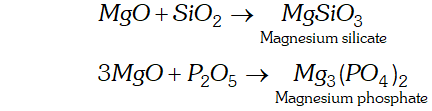Q. What is a mineral ? How does it differ from an ore ?
Ans. The natural materials or the chemical compounds in which the metals occur in the earth are called minerals. The mineral from which the metal is conveniently and economically extracted is called an ore. Thus, all ores are minerals but all minerals are not $c$
Q. Pine oil is generally added in the Froth Floatation process. Explain.
Ans. In the froth floatation process, sulphide ore is to be separated from gangue or earthy impurities. The impure ore is mixed with water and pine oil in a tank and steam is passed through it. Now, gangue particles are wetted by water while the ore by oil. With the help of steam, the sulphide ore rises in the form of a foam while the gangue impurities settle at the bottom of the container.
Q. Aluminium metal is generally used for the extraction of chromium and manganese from their oxide ores. Explain.
Ans. Aluminium has a very strong affinity for oxygen. It reduces the oxides of chromium and manganese to the metallic forms. It can also be explained on the basis of Ellingham diagram.Q. Why partial roasting of sulphide ore is done in the metallurgy of copper ?
Ans. Partial roasting of sulphide ore is carried in order to convert it to the oxide form. The oxide form then reduces the remaining sulphide ore to the metallic form. This is called auto reduction.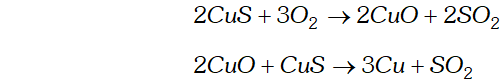Q. What is thermit process ? What is its utility ?
Ans. Aluminium metal is a powerful reducing agent. It reduces the oxides of metals like iron and chromium to the metallic state upon heating.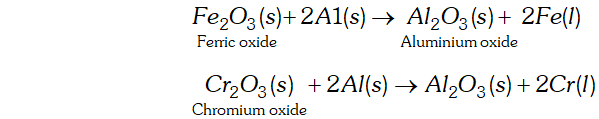The reaction is known as thermit reaction. It is highly exothermic in nature. As a result, the metal iron or chromium is in the molten state. It can be used to fill the cracks in the railway tracks and also the cracks in the machine parts. This process is known as thermit process.
Q. The value of $\Delta_{f} G^{\circ}$ for formation of $C r_{2} O_{3}$ is $-540 k J m o r^{-l}$ and that of $A l_{2} O_{3}$ is $-827 k J \mathrm{mol}^{-1} .$ Is the reduction of $\mathrm{Cr}_{2} \mathrm{O}_{3}$ possible with aluminium?
Ans. The two equations are: $\frac{4}{3} A l(s)+O_{2}(g) \rightarrow \frac{2}{3} A l_{2} O_{3}(s) ; \Delta_{f} G_{A l, A_{2} O_{3}}^{\circ}=-827 k J m o l^{1} \ldots$ $\frac{4}{3} C r(s)+O_{2}(g) \rightarrow \frac{2}{3} C r_{2} O_{3}(s) ; \Delta_{f} G^{\circ}=-540 k J m o l^{1}$ Subtracting Eq. (ii) from Eq. (i), we have, $\frac{4}{3} A l(s)+\frac{2}{3} C r_{2} O_{3}(s) \rightarrow \frac{2}{3} A l_{2} O_{3}(s)+\frac{4}{3} C r(s) ; \Delta_{r} G^{\circ}$ $$=-287 \mathrm{kJ} \mathrm{mol}^{-1}$$ since $\Delta_{r} G^{\circ}$ of the combined redox reaction is $-v e$ Therefore reduction of $C r_{2} O_{3}$ by $A l$ is possible.
Q. Zinc and not copper is used for the recovery of silver from the complex $\left[A g(C N)_{2} J^{-}\right.$ Discuss.
Ans. Zinc is a very powerful reducing agent and readily displaces silver present in the complex.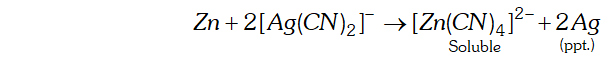No doubt copper is also more reducing than silver but is not so effective as zinc. Moreover, zinc is cheaper than copper.
Q. What is the role of cryolite in the metallurgy of aluminium ?
Ans. The role of cryolite is two-fold. (i) It makes alumina a good conductor of electricity. (i) It lowers the fusion (melting point) temperature of the bath from $2323 K$ to about $1140 K$
Q. What is Van Arkel method of obtaining ultrapure metals ?
Ans. The impure metal (Ti or Zr) is first converted into its volatile ¡odide by heating with $I_{2}$ at some low temperature and then decomposing the volatile iodide at a suitable high temperature to give the pure metal. For example,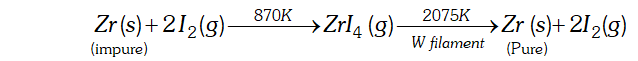Q. What is a slag?
Ans. A slag is an easily fusible material which is formed when gangue still present in the roasted ore the calcined ore combines with the flux For example, in the metallurgy of iron, $\mathrm{CaO}(\mathrm{Flux})$ combines with silica gangue to form an easily fusible calcium silicate $\left(C a S i O_{3}\right)$ slag. $\mathrm{CaCO}_{3} \rightarrow \mathrm{CaO}+\mathrm{CO}_{2} ; \mathrm{CaO}+\mathrm{SiO}_{2} \rightarrow \mathrm{CaSiO}_{3}(\mathrm{slag})$
Q. What is the significance of leaching in the extraction of aluminium ?
Ans. - Aluminium usually contains silica $\left(\mathrm{SiO}_{2}\right),$ iron oxide and titanium oxide $\left(T i O_{2}\right)$ as impurities. These impurities can be removed by the process of leaching. During leaching, the powdered bauxite ore is heated with a concentrated $(45 \%)$ solution of $N a O H$ at $473-523 K,$ when alumina dissolves as sodium meta-aluminate and silica $\left(\mathrm{Si} \mathrm{O}_{2}\right)$ as sodium silicate leaving the impurities behind.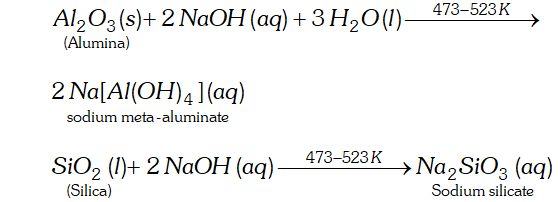The impurities are filtered off and the solution of sodium meta-aluminate is neutralized by passing $\mathrm{CO}_{2}$ when hydrated alumina separates out while sodium silicate remains in the solution. The hydrated alumina thus obtained is filtered, dried and heated to give back pure alumina.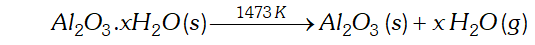Thus, the significance of leaching in the extraction of aluminium is to prepare pure alumina from the bauxite ore.
Q. At a site, low grade copper ores are available and zinc and iron scraps are also available. Which of the two scraps will be more suitable for reducing the leached copper ore and why?
Ans. The $E^{\circ}$ value for the redox couple $Z n^{2+} / Z n(-0.76 V)$ is more negative than that of $F e^{2+} / F e(-0.44 V)$ redox couple. Therefore, zinc is more reactive than iron and hence reduction will be faster in case if zinc scraps are used. But zinc is a costlier metal than iron so using iron scraps would be more economical.
Q. Why is the extraction of copper from pyrite difficult than that from its oxide ore through reduction ?
Ans. Carbon is a poor reducing agent for sulphide ores whereas it is good reducing agent for oxide ores.
Q. Why copper matte is put in silica lined converter ?
Ans. Copper matte contains $\mathrm{Cu}_{2} \mathrm{S}$ and $\mathrm{FeS.}$. In the converter FeS gets converted into $F e O$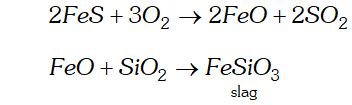Q. Differentiate between “minerals” and “ores”
Ans. . The naturally occurring chemical substances in form of which the metals occur in the earth along with impurities are called minerals. The mineral from which the metal can be extracted conveniently and economically is called an ore. Thus, all ores are minerals but all minerals are not ores. For example, iron is found in the earth's crust as oxides, carbonates and sulphides. Out of these minerals of iron, the oxides of iron are employed for extraction of the metal. Therefore, oxides of iron are the ores of iron. Similarly, aluminium occurs in earth's crust in form of two minerals, i.e., bauxite $\left(A l_{2} O_{3} . x H_{2} O\right)$ and clay $\left(A l_{2} O_{3} .2 \mathrm{SiO}_{2} .2 \mathrm{H}_{2} \mathrm{O}\right) .$ Out of these two minerals, Al can be conveniently and economically extracted from bauxite. Therefore, bauxite is the ore of aluminium.
Q. Write one point of difference between electrolytic reduction and reduction with carbon. Give one example of each.
Ans. - The electrolytic reduction takes place at the cathode by the gain of electrons in electrolysis. At the same time, carbon reduction is carried by heating a metal oxide with coke. For example,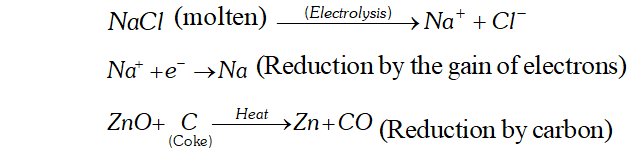Q. Why is the extraction of copper from pyrite difficult than that from its oxide ore through reduction ?
Ans. - The standard free energy $\left(\Delta_{f} G^{\circ}\right)$ of formation of $C u_{2} S$ is greater than those of $C S_{2}$ and $H_{2} S\left(C S_{2}\right.$ is, infact, an endothermic compound). Therefore, neither carbon nor hydrogen can reduce to $\mathrm{Cumetal.}$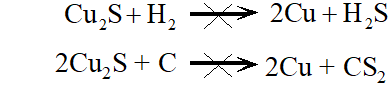In contrast, $\Delta_{f} G^{\circ}$ of $C u_{2} O$ is much lower than that of $C O_{2}$ and hence carbon can easily reduce to $\mathrm{Cu}_{2} \mathrm{O}$ to $\mathrm{Cu}$ $\mathrm{Cu}_{2} \mathrm{O}(\mathrm{s})+\mathrm{C}(\mathrm{s}) \rightarrow 2 \mathrm{Cu}(\mathrm{s})+\mathrm{CO}(\mathrm{g})$ It is because of this reason that the extraction of copper from pyrite is difficult than from its oxide ore through reduction.
Q. Giving examples, differentiate between 'roasting,' and "calcination'.
Ans. The process of converting carbonates and hydroxide ores of metals to their respective oxides by heating them strongly below their melting points either in absence or limited supply of air is called calcination. For example,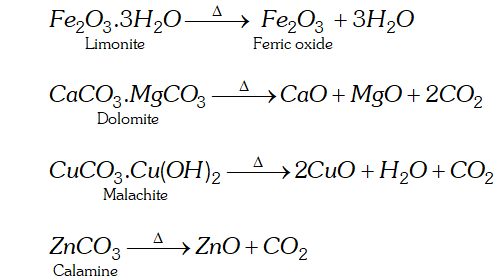On the other hand, the process of converting a sulphide ore into its metallic oxide by heating strongly below its melting point in excess of air is called roasting. For example,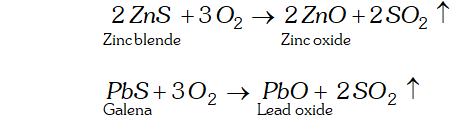Q. Why copper matte is put in silica lined converter ?
Ans. Copper matte chiefly consists of $\mathrm{Cu}_{2} \mathrm{S}$ along with some unchanged FeS. when a blast of hot air is passed through molten matte taken in a silica lined converter, FeS present in matte is oxidised to $F e O$ which combines with silica $\left(S i O_{2}\right)$ to form $\mathrm{FeSiO}_{3}$ slag.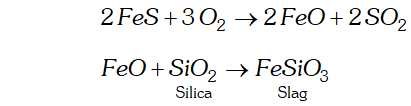When whole of iron has been removed as slag, some of the undergoes oxidation to form which then reacts with more to form copper metal. Thus, copper matte is heated in silica lined converter to remove FeS present in matte as slag.
Q. Write chemical reactions taking place in the extraction of zinc from zinc blende.
Ans. The various steps involved are (a) Concentration. The ore is crushed and then concentrated by froth-floatation process. (b) Roasting. The concentrated ore is then roasted in presence of excess of air at about $1200 \mathrm{K}$ when zinc oxide $(\mathrm{ZnO})$ is formed.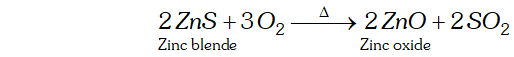(c) Reduction. Zinc oxide obtained above is mixed with powdered coke and heated to $1673 \mathrm{K}$ in a fire clay retort when it is reduced to zinc metal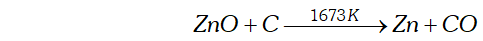At $1673 K, \text { zinc metal being volatile (b.p. } 1180 \mathrm{K}),$ distils over and is condensed. (d) Electrolytic refining. Impure zinc is made the anode while cathode consists of a sheet of pure zinc. The electrolyte consists of $Z n S O_{4}$ solution acidified with dil. $H_{2} S O_{4} .$ On passing electric current, pure $Z n$ gets deposited on the cathode.
Q. Name the process from which chlorine is obtained as a byeproduct. what will happen if an aqueous solution of $N a C l$ is subjected to electrolysis?
Ans. Sodium metal is prepared by Down's process. It involves the electrolysis of a fused mixture of $N a C l$ and $C a C l_{2}$ at $873 K$ During electrolysis, sodium is discharged at the cathode while $\mathrm{Cl}_{2}$ is obtained at the anode as a bye-product.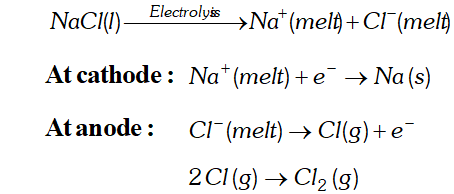If, however, an aqueous solution of $N a C l$ is electrolysed, $H_{2}$ is evolved at the cathode while is obtained at the anode. The reason being that the $E^{\circ}$ of redox couple is much lower than that of and hence water is reduced to in preference to ions. $\mathrm{NaOH}$ is, however, obtained in the solution.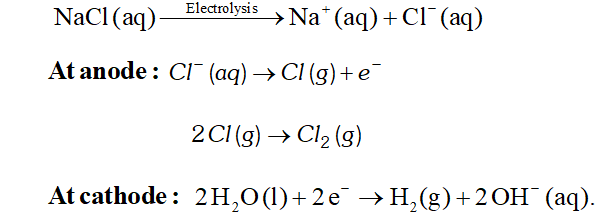Q. What is the role of graphite in the electrometallurgy of aluminium ?
Ans. In the electrometallurgy of alumina, a fused mixture of alumina, cryolite and fluorspar $\left(\mathrm{CaF}_{2}\right)$ is electrolysed using graphite as anode and graphite lined iron as cathode. During electrolysis, $A l$ is liberated at the cathode while $C O$ and $C O_{2}$ are liberated at the anode. At cathode : $A I^{3+}(\text { melt }) \rightarrow A l(l)$ $\begin{array}{ll}{\text { Atanode: }} & {C(s)+O^{2-}(\text {melt}) \rightarrow C O(g)+2 e^{-}} \\ {} & {C(s)+2 O^{2-}(\text {melt}) \rightarrow C O_{2}(g)+4 e^{-}}\end{array}$ If instead of graphite, some other metal is used as the anode, then $O_{2}$ liberated will not only oxidise the metal of the electrode but would also convert some of the Alliberated at the cathode back to $A l_{2} O_{3}$ since graphite is much cheaper than any metal, therefore, graphite is used as the anode. Thus, the role of graphite in electrometallurgy of Al is to prevent the liberation of at the anode which may otherwise oxidise some of the liberated Al back to $\mathrm{Al}_{2} \mathrm{O}_{3}$
Q. Describe a method for refining nickel.
Ans. Mond process: It is used for refining of nickel. When impure nickel is heated in a current of $C O$ at $330-350 K,$ it forms volatile nickel tetracarbonyl complex leaving behind the impurities. The nickel tetracarbonyl thus obtained is then heated to a higher temperature $(450-470 K)$ when it undergoes thermal decomposition giving pure nickel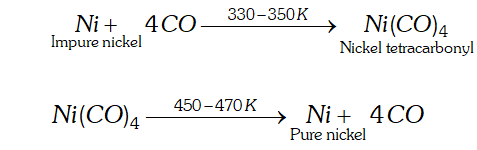Q. Predict conditions under which $A l$ might be expected to reduce $M g O$
Ans. Aluminium can reduce magnesium oxide at a temperature of about $2000 K$ because in this temperature range the line of $\Delta G^{\ominus}_{\left(M g, M_{g} O\right)}$ lies above the line of $\Delta G_{\left(A l, A l_{2} O_{3}\right)}^{\Theta}$ in the Elingham diagram. Therefore, under these condition $\Delta_{r} G^{\ominus}$ for reduction of $M g O$ with $A l$ will be negative and hence the reaction, $3 \mathrm{MgO}+2 \mathrm{Al} \rightarrow 3 \mathrm{Mg}+\mathrm{Al}_{2} \mathrm{O}_{3}$ would be feasible.
Q. Explain the following: (a) Carbon reduction process is not applied for reducing aluminium oxide to aluminium. (b) Aqueous solution of sodium chloride cannot be used for the isolation of sodium by electrolytic reduction method. (c) Roasting is quite helpful to convert sulphide ore of a metal to the oxide form. (d) Thermit process is quite useful for repairing the broken parts of a machine.
Ans. (a) Carbon reduction process is not applied for reducing aluminium oxide to aluminium. Actually, aluminium metal itself a very powerful reducing agent and can easily take up oxygen from the oxides of metals like iron, manganese, chromium etc. Therefore, it cannot be reduced by carbon. (b) If an aqueous solution of sodium chloride is used, then sodium metal which is formed at cathode will immediately react with water. Since sodium has a very strong affinity for water, the reaction will be highly exothermic and the metal will catch fire. (c) Roasting is carried by heating the metal sulphide with excess of oxygen or air. As a result the metal sulphide will be oxidised to its oxide. For example,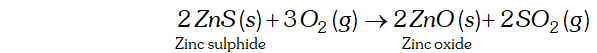(d) In the thermit process, oxides of metals like iron are reduced by aluminium. The reaction is highly exothermic and a large amount of heat is evolved in the reaction. As a result, the metal will be in the molten state. For example,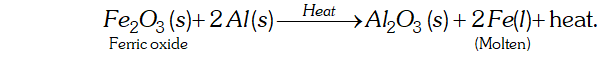If the molten metal is allowed to fall between the broken parts of a machine, the gaps will be filled up and the machine will be repaired.
Q. Write down the reactions taking place in different zones in the blast furnace during the extraction of iron.
Ans. Following reactions occur in the blast furnace (i) Zone of combustion: Near the tuyeres, coke burns to form carbon dioxide $$C+O_{2} \rightarrow C O_{2} ; \Delta H=-393.3 k_{U}$$ since the reaction is exothermic, lot of heat is produced and the temperature here is around $2170 K$ (ii) Zone of heat absorption: This is lower part of the Furnace and the temperature here is between $1423-1673 \mathrm{K.As} \mathrm{CO}_{2}$ formed near tyres moves up; it meets the descending charge. The coke present in the charge reduces $\mathrm{CO}_{2}$ to $\mathrm{CO}$ $\mathrm{CO}_{2}+\mathrm{C} \rightarrow 2 \mathrm{CO} ; \Delta \mathrm{H}=+163.2 \mathrm{kJ}$ since this reaction is endothermic, therefore the temperature gradually falls to $1423 \mathrm{K}$ (iii) Zone of slag formation: It is the middle part of the furnace. The temperature here is around $1123 \mathrm{K} .$ In this region, limestone decomposes to form $\mathrm{CaO}$ and $\mathrm{CO}_{2} .$ The $\mathrm{CaO}$ thus formed acts as a flux and combines with silica (Present as an impurity) to form fusible calcium silicate slag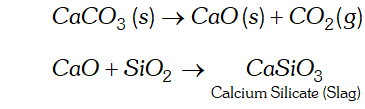(iv) Zone of reduction: This is the upper part of the furnace. The temperature here is around $823 \mathrm{K} .$ Here the ores are reduced to $\mathrm{FeOby} \mathrm{CO}$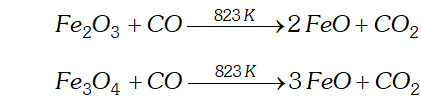But the further reduction of $F e O$ to $F e$ by $C O$ occur around $1123 K$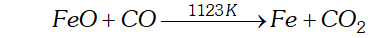However, direct reduction of iron ores, i.e. haematite, magnetite etc, which might have escaped reduction around $823 \mathrm{K}$, gets completely reduced to iron by carbon above $1123 \mathrm{K}$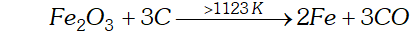(v) Zone of fusion : This is the lower part of the furnace. Temperature here is in between $1423-1673 K .$ In this region, spongy iron melts and dissolves some $C, S, P, S i, M n,$ etc. slag also melts in this region. Both the molten slag and the molten iron trickle down into hearth where they form two separate layers. The molten slag being lighter forms the upper layer while molten iron being heavier form the lower layer. The two liquids are periodically trapped off. The iron thus obtained from the furnace contains about $4 \%$ carbon and many impurities in smaller amount (e.g., S, P, Si, Mn). This is called pig iron and is cast into variety of shapes. Cast iron is different from pig iron and is made by melting pig iron with scrap iron and coke using hot air blast. It has slightly lower carbon content (about $3 \%$ ) is extremely hard and brittle
Q. How can you separate alumina from silica in bauxite ore associated with silica ? Give equations, if any.
Ans. Pure alumina may be separated from bauxite by Baeyer's process as discussed below. The bauxite ore associated with silica is heated with a concentrated solution of $N a O H$ at 473 - $523 \mathrm{Kand} 35-36$ bar pressure. Under these conditions, alumina dissolves as sodium meta-aluminate and silica as sodium silicate leaving behind the impurities.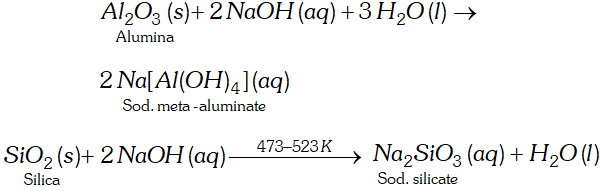The resulting solution is filtered to remove the undissolved impurities, if any and neutralized by passing $\mathrm{CO}_{2}$ gas. Thereafter, the solution is seeded with freshly prepared samples of hydrated alumina when hydrated alumina gets precipitated leaving sodium silicate in the solution.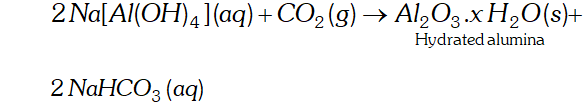The hydrated alumina thus precipitated is filtered, dried and heated to give back pure $A l_{2} O_{3} .$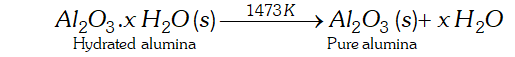Alternatively, serpeck's process may be employed for the purification of white bauxite which contains silica as the impurity. The ore is powdered, mixed with coke and heated to $2073 \mathrm{Kin}$ an atmosphere of $N_{2}$ gas. Alumina combines with to form aluminium nitride while silica is reduced to silicon which volatilizes off at this temperature.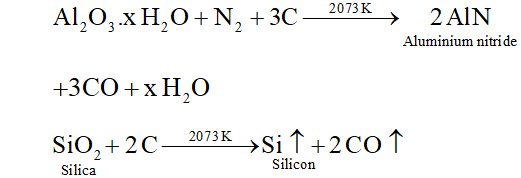Aluminium nitride thus obtained is hydrolysed by water to form aluminium hydroxide which, when ignited, gives pure alumina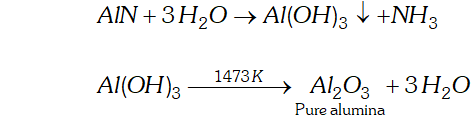This process has one distinct advantage that ammonia is obtained as a by product.
Q. Outline the principles of refining of metals by the following methods (i) Zone refining (ii) Electrolytic refining (iii) Vapour phase refining
Ans. (i) Zone refining : As that of Ans. of Q. no.-4. (ii) Electrolytic refining: A large number of metals such as copper, silver, gold, lead, nickel, chromium, zinc, aluminium etc are refined by this method. In this method the impure metal is converted into a block which forms the anode while cathode is made up of a pure strip of the same metal. These electrodes are suspended in an electrolyte which is the solution of a soluble salt of the metal usually a double salt of the metal. When electric current is passed, metal ions from the electrolyte are deposited at the cathode in the form of pure metal while an equivalent amount of metal dissolves from the anode and goes into the electrolyte solution as metal ions. The process takes place as under Anode : $M \rightarrow M^{n+}+n e^{-}$ Cathode : $M^{n+}+n e^{-} \rightarrow M$ The net result is the transfer of pure metal from the anode to the cathode. The voltage applied for electrolysis is such that the impurities of more basic metals (more electropositive metals) remain in the solution as ions whereas impurities of the less basic metals (Less electropositive metals) settle down under the anode as anode mud or anode sludge (iii) Vapour - phase refining: In this method, the crude metal is freed from impurities by first converting it into a suitable volatile compound by heating it with a specific reagent at a lower temperature and then decomposing the volatile compound at some higher temperature to give the pure metal. Thus, the two requirements are: (1) The metal should form a volatile compound with a suitable reagent. (2) The volatile compound should be easily decomposable so that the recovery is easy.

#### Joseph - Oct. 23, 2020, 11:04 a.m.

This question are OK but.l need questions in number order.

#### M.PRATHOSH - Sept. 10, 2020, 8:29 a.m.

First if you change tha (Q)to numbers .it is easy way to find the questions .

#### Sai sharanya - July 11, 2020, 4:36 p.m.

Can we get some questions on Ellingham diagram

#### kadesh - May 10, 2020, 7:43 a.m.

thank you

None
Free Study Material